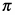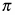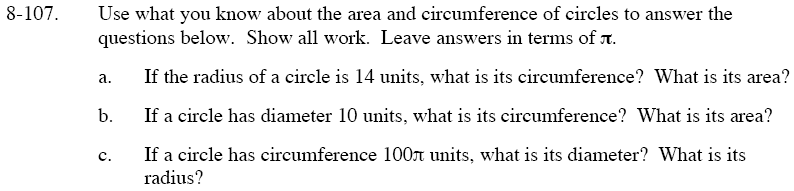Home > GC > Chapter 8 > Lesson 8.3.3 > Problem8-107

8-107.
1. Use what you know about the area and circumference of circles to answer the questions below. Show all work. Leave answers in terms of. Homework Help ✎

1. If the radius of a circle is 14 units, what is its circumference? What is its area?

2. If a circle has diameter 10 units, what is its circumference? What is its area?

3. If a circle has circumference 100units, what is its diameter? What is its radius?Remember that the area of a circle is πr², while the circumference of a circle is 2πr.

Area = 196π
Circumference = 28π